/

### The generalised extreme value distribution

The generalised extreme value (or generalized extreme value) distribution characterises the behaviour of ‘block maxima’ under certain (somewhat restrictive) regularity conditions. See also Nematrian’s webpages about Extreme Value Theory (EVT).

 Distribution name Generalised extreme value (GEV) distribution (for maxima) Common notation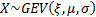Parameters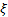= shape parameter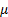= location parameter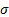= scale parameter Domain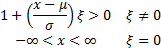Probability density function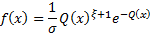where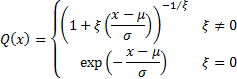Cumulative distribution function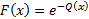Mean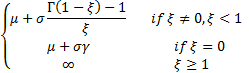where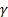is Euler’s constant, i.e.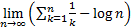Variance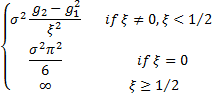Where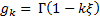Skewness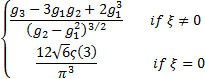where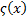is the Riemann zeta function, i.e.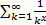. (Excess) kurtosis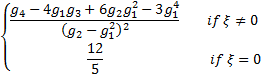Other commentsdefines the tail behaviour of the distribution. The sub-families defined by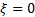(Type I),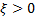(Type II) and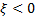(Type III) correspond to the Gumbel, Frechét and Weibull families respectively.   An important special case when analysing threshold exceedances involves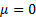(and normally) and this special case may be referred to as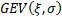.

Nematrian web functions

Functions relating to the above distribution may be accessed via the Nematrian web function library by using a DistributionName of “gev”. For details of other supported probability distributions see here.